Next: Model order selection Up: tr02cb1 Previous: Uniqueness

# Maximum Likelihood estimation

Throughout the remainder of this paper, we are going to keep the parameter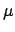fixed at its ML estimate: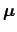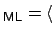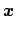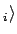(assuming zero-mean sources) and will assume that it has been removed from the data. The mean can always be reintroduced after model estimation using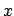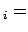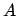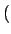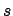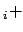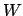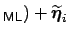, where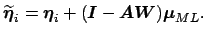Without loss of generality we will also assume that the sources have unit variance for we can freely exchange arbitrary scaling factors between the source signals and the associated columns of the mixing matrix.

If the noise covariance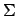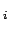was known we can use its Cholesky decomposition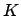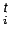to rewrite equation 2: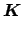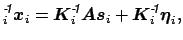and obtain a new representation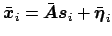where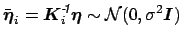, i.e. where the noise covariance is isotropic at every voxel location. During this step, the data are pre-whitened with respect to the noise covariance, which is not to be confused with (spatial) pre-whitening of the data matrix using singular-value decomposition. In order to simplify further notation, we are therefore going to assume that the data contain isotropic noise and drop the bars.

Noise and signal are assumed to be uncorrelated and therefore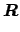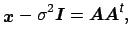where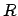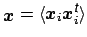denotes the covariance matrix of the observations. Letbe the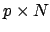matrix of voxel-wise pre-whitened data vectors and let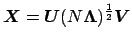be its singular value decomposition. Furthermore let the rank of the mixing matrix, i.e. the number of source processes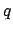, be known. Then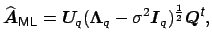(5)

where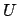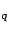and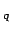contain the firsteigenvectors and eigenvalues ofandand where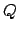denotes a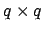orthogonal rotation matrix.

Estimating the mixing matrixthus reduces to identifying the square matrixafter whitening the data with respect to the noise covarianceand projecting the temporally whitened observations onto the space spanned by theeigenvectors of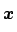with largest eigenvalues. From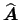, the maximum likelihood source estimates are obtained using generalised least squares: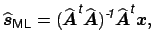(6)

and the ML estimate of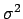becomes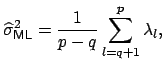(7)

i.e. is the average of the eigenvalues in the minor subspace spanned by the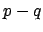smallest eigenvectors. Solving the model in the case of a full noise covariance where the noise covariance is unknown can be achieved by iterating estimates forand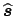and re-estimating the noise covariances from the residuals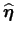. The estimation process in the presence of non-isotropic noise is computationally much more involved than estimation in the standard noise free setting. The form ofneeds to be constrained, e.g. we can use the common approaches to FMRI noise modelling [Bullmore et al., 1996,Woolrich et al., 2001], and restrict ourselves to autoregressive noise. However, since the exploratory approach allows modelling of various sources of variability, e.g. temporally consistent physiological noise, as part of the signal in equation 2, the noise model itself can actually be quite simplistic. Estimation offrom residuals in the case of autocorrelated noise is discussed in detail in [Woolrich et al., 2001] which is the approach used in this case.

The maximum likelihood solutions given in equations 5-7 give important insight into the methodology. Firstly, in the case whereandare known, the maximum likelihood solution foris contained in the principal eigenspace ofof dimension, i.e. the span of the firsteigenvectors equals the span of the unknown mixing matrix. Projecting the data onto the principal eigenvectors is not just a convenient technique to deal with the high dimensionality in FMRI data but is part of the maximum likelihood solution under the sum of square loss. Even if estimation techniques are employed that do not use an initial PCA step as part of the ICA estimation, the final solution under this model is necessarily contained in the principal subspace. Secondly, combining these results with the uniqueness results stated earlier we see that only in the case where the analysis is performed in the appropriate lower-dimensional subspace of dimensionare the source processes uniquely identifiable. Finally, equations 5-7 imply that the standard noise-free ICA approach with dimensionality reduction using PCA implicitly operates under an isotropic noise model.

The remainder of this paper illustrates that by making this specific noise model explicit in the modelling and estimation stages, we can address important questions of model order selection, estimation and inference in a consistent way.

An immediate consequence of the fact that we are operating under an isotropic noise model is that as an initial pre-processing step we will modify the original data time courses to be normalised to zero mean and unit variance. This appears to be a sensible step in that on the one hand we know that the voxel-wise standard deviation of resting state data varies significantly over the brain but on the other hand, all voxels' time courses are assumed to be generated from the same noise process. This variance-normalisation pre-conditions the data under the 'null hypotheses' of purely Gaussian noise, i.e. in the absence of any signal: the data matrix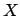is identical up to second order statistics to a simple set of realisations from a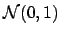noise process. Any signal component contained inwill have to reveal itself via its deviation from Gaussianity. This will turn out to be of prime importance both for the estimation of the number of sources and the final inferential steps.

After a voxel-wise normalisation of variance, two voxels with comparable noise level that are modulated by the same signal time course,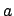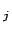say, but by different amounts will have the same regression coefficient upon regression against. The difference in the original amount of modulation is therefore contained in the standard deviation of the residual noise. Forming voxel-wise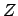statistics, i.e. dividing the PICA maps by the estimated standard deviation of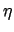, thus is invariant under the initial variance-normalisation.

SubsectionsNext: Model order selection Up: tr02cb1 Previous: Uniqueness
Christian F. Beckmann 2003-08-05